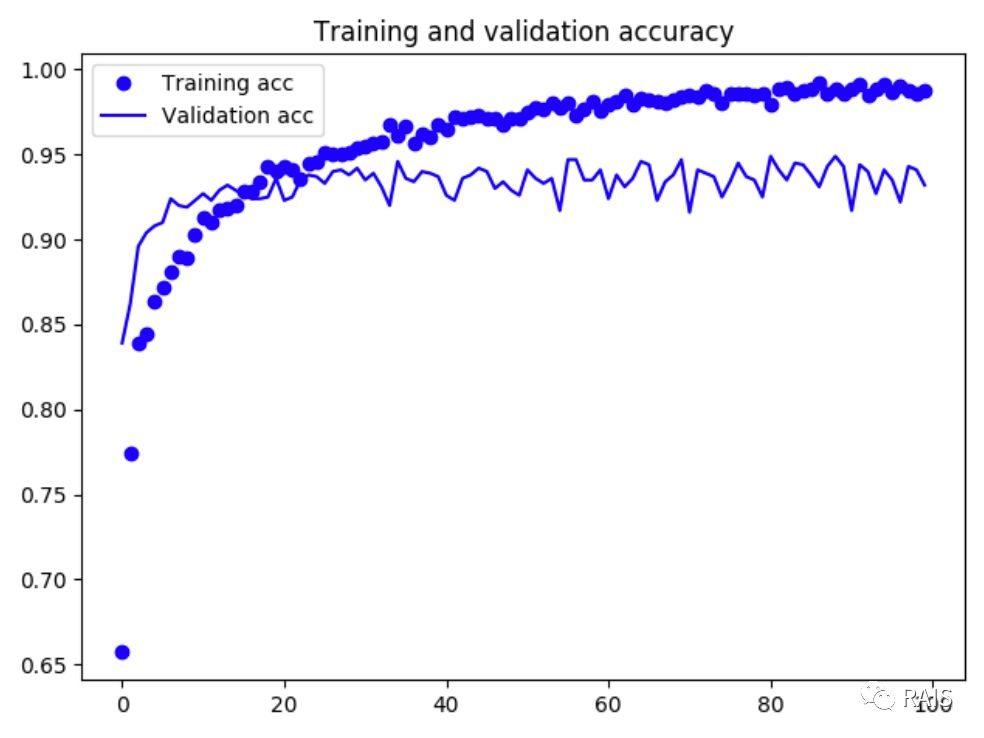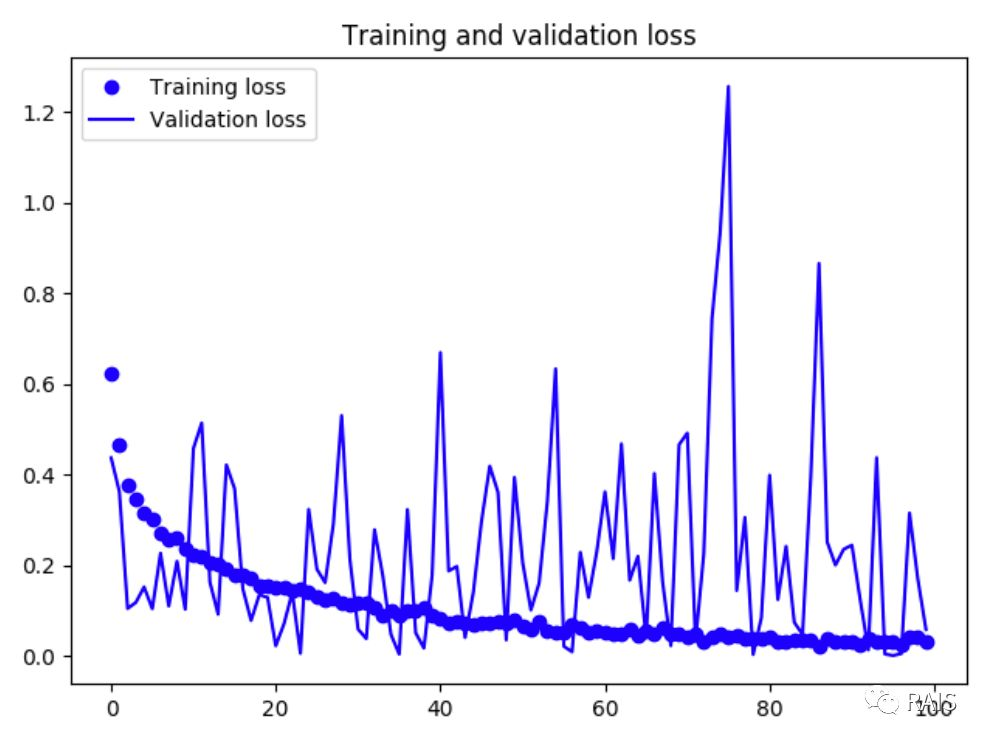# AI：拿来主义——预训练网络（二）

2019/04/10 10:10

1. 在已经训练好的网络（基网络）基础上，添加自定义的层；

2. 冻结基网络并训练新添加的层；

3. 冻结基网络的一部分层，另一部分可训练；

4. 联合训练解冻的这些层和添加的部分。

conv_base.trainable = True
set_trainable = False
for layer in conv_base.layers:
if layer.name == 'layer_name':
set_trainable = True
if set_trainable:
layer.trainable = True
else:
layer.trainable = False1. 计算机视觉领域中，卷积神经网络的表现非常不错，并且在数据集较小的情况下，表现让人是非常优秀的。

2. 数据增强是很好的避免过拟合的方法，过拟合产生的主要原因可能是数据量太少或者是参数过多。

3. 特征提取可以比较好的将现有的神经网络应用于小型数据集，还可以使用微调的方式进行优化。

#!/usr/bin/env python3
​
import os
import time
​
import matplotlib.pyplot as plt
from keras import layers
from keras import models
from keras import optimizers
from keras.applications import VGG16
from keras.preprocessing.image import ImageDataGenerator
​
​
def cat():
base_dir = '/Users/renyuzhuo/Desktop/cat/dogs-vs-cats-small'
train_dir = os.path.join(base_dir, 'train')
validation_dir = os.path.join(base_dir, 'validation')
​
train_datagen = ImageDataGenerator(
rescale=1. / 255,
rotation_range=40,
width_shift_range=0.2,
height_shift_range=0.2,
shear_range=0.2,
zoom_range=0.2,
horizontal_flip=True,
fill_mode='nearest')
​
test_datagen = ImageDataGenerator(rescale=1. / 255)
​
train_generator = train_datagen.flow_from_directory(
train_dir,
target_size=(150, 150),
batch_size=20,
class_mode='binary')
​
validation_generator = test_datagen.flow_from_directory(
validation_dir,
target_size=(150, 150),
batch_size=20,
class_mode='binary')
​
# 定义密集连接分类器
conv_base = VGG16(weights='imagenet',
include_top=False,
input_shape=(150, 150, 3))
conv_base.trainable = True
set_trainable = False
for layer in conv_base.layers:
if layer.name == 'block5_conv1':
set_trainable = True
if set_trainable:
layer.trainable = True
else:
layer.trainable = False
model = models.Sequential()
model.add(layers.Dense(256, activation='relu', input_dim=4 * 4 * 512))
​
conv_base.summary()
​
# 对模型进行配置
model.compile(loss='binary_crossentropy',
optimizer=optimizers.RMSprop(lr=1e-5),
metrics=['acc'])
​
# 对模型进行训练
history = model.fit_generator(
train_generator,
steps_per_epoch=100,
epochs=100,
validation_data=validation_generator,
validation_steps=50)
​
# 画图
acc = history.history['acc']
val_acc = history.history['val_acc']
loss = history.history['loss']
val_loss = history.history['val_loss']
epochs = range(len(acc))
plt.plot(epochs, acc, 'bo', label='Training acc')
plt.plot(epochs, val_acc, 'b', label='Validation acc')
plt.title('Training and validation accuracy')
plt.legend()
plt.show()
plt.figure()
plt.plot(epochs, loss, 'bo', label='Training loss')
plt.plot(epochs, val_loss, 'b', label='Validation loss')
plt.title('Training and validation loss')
plt.legend()
plt.show()
​
​
if __name__ == "__main__":
time_start = time.time()
cat()
time_end = time.time()
print('Time Used: ', time_end - time_start)### 作者的其它热门文章

0
0 收藏

0 评论
0 收藏
0